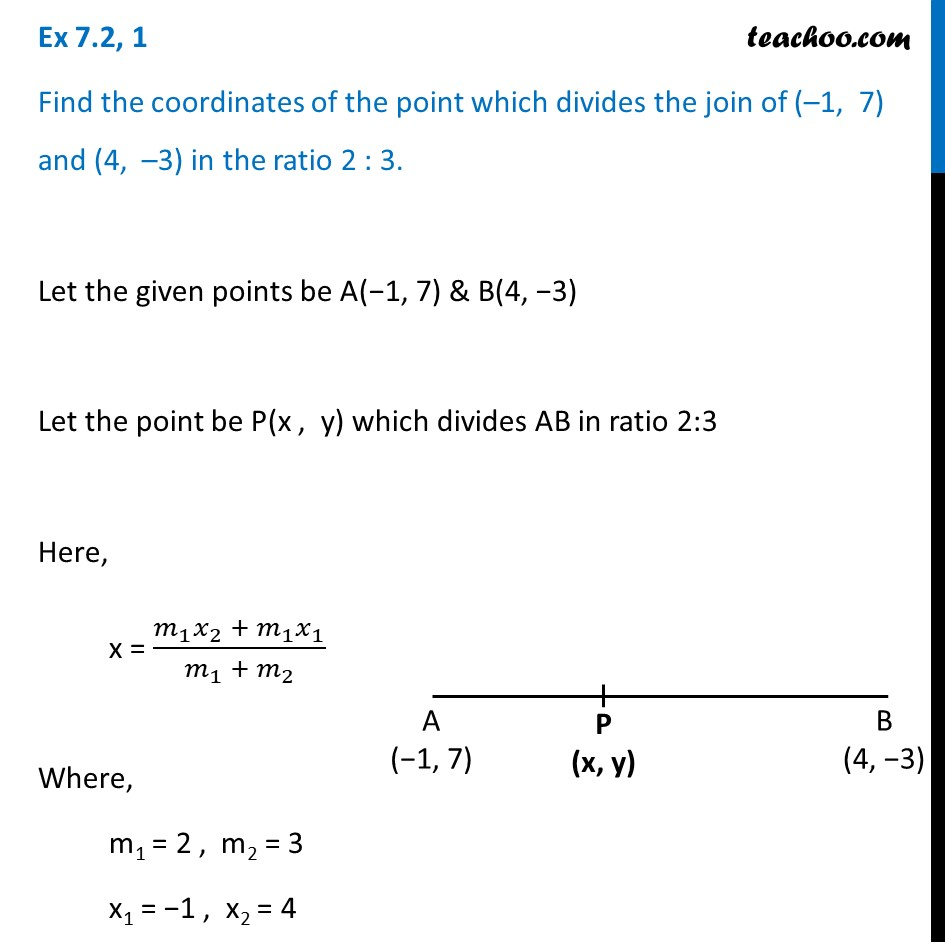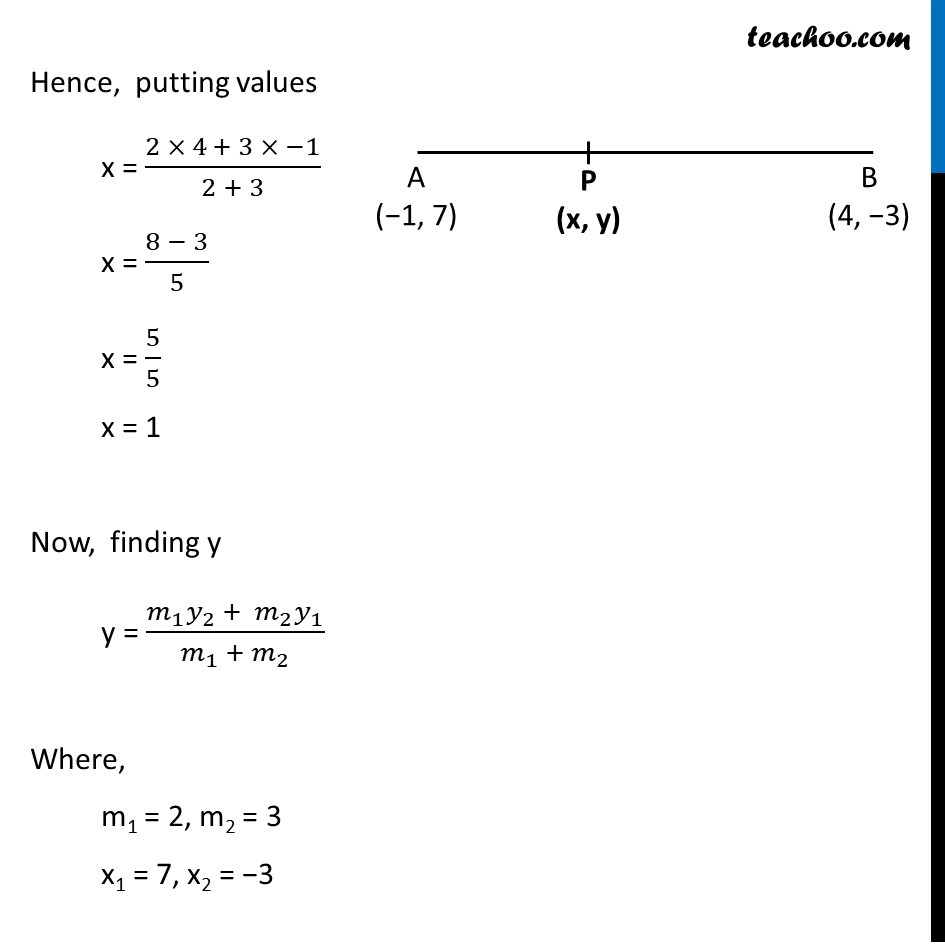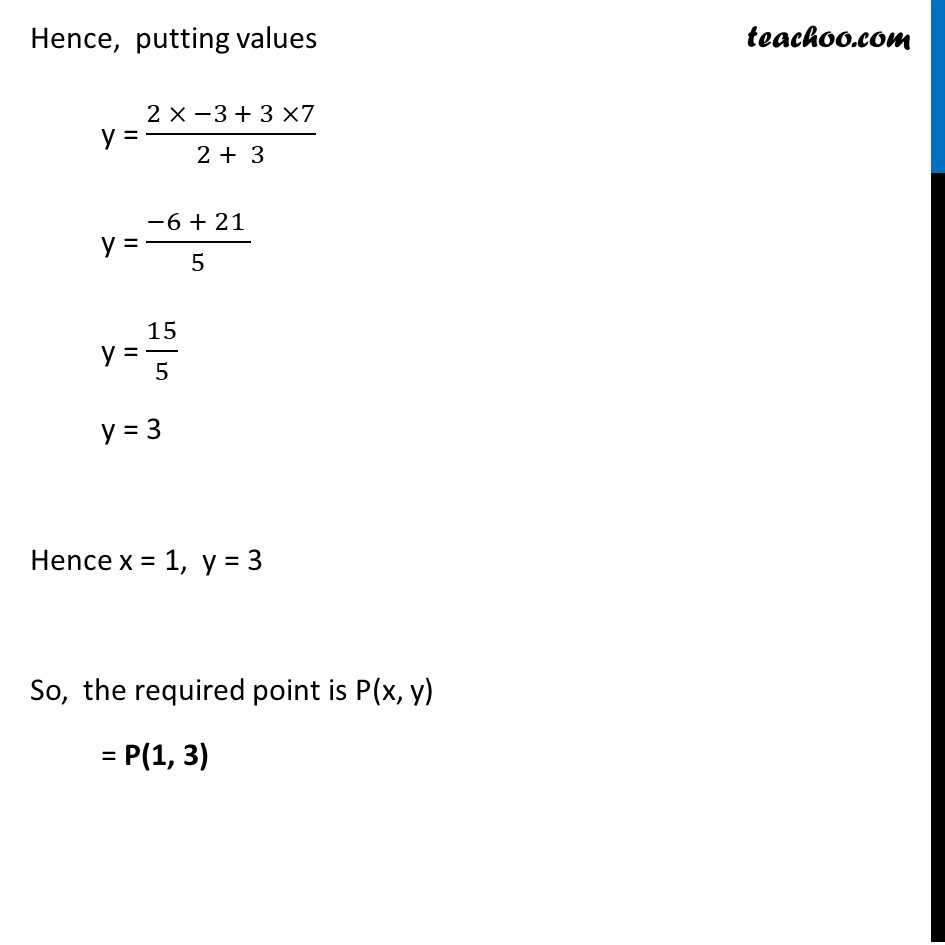Chapter 7 Class 10 Coordinate Geometry

Class 10
Important Questions for Exam - Class 10Introducing your new favourite teacher - Teachoo Black, at only ₹83 per month

### Transcript

Ex 7.2, 1 Find the coordinates of the point which divides the join of (–1, 7) and (4, –3) in the ratio 2 : 3. Let the given points be A(−1, 7) & B(4, −3) Let the point be P(x , y) which divides AB in ratio 2:3 Here, x = (𝑚_1 𝑥_2 + 𝑚_1 𝑥_1)/(𝑚_1 + 𝑚_2 ) Where, m1 = 2 , m2 = 3 x1 = −1 , x2 = 4 Hence, putting values x = (2 × 4 + 3 × −1)/(2 + 3) x = (8 − 3)/5 x = 5/5 x = 1 Now, finding y y = (𝑚_1 𝑦_2 + 𝑚_2 𝑦_1)/(𝑚_(1 )+ 𝑚_2 ) Where, m1 = 2, m2 = 3 x1 = 7, x2 = −3 Hence, putting values y = (2 × −3 + 3 ×7)/(2 + 3) y = (−6 + 21 )/5 y = 15/5 y = 3 Hence x = 1, y = 3 So, the required point is P(x, y) = P(1, 3)